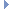OpenMM
MonteCarloBarostat Class Reference

This class uses a Monte Carlo algorithm to adjust the size of the periodic box, simulating the effect of constant pressure. More...Inheritance diagram for MonteCarloBarostat:

List of all members.

## Public Member Functions

def Pressure
Pressure() -> std::string const &.
def getDefaultPressure
getDefaultPressure(self) -> double
def setDefaultPressure
Set the default pressure acting on the system.
def getFrequency
getFrequency(self) -> int
def setFrequency
Set the frequency (in time steps) at which Monte Carlo pressure changes should be attempted.
def getTemperature
getTemperature(self) -> double
def setTemperature
Set the temperature at which the system is being maintained.
def getRandomNumberSeed
getRandomNumberSeed(self) -> int
def setRandomNumberSeed
Set the random number seed.
def usesPeriodicBoundaryConditions
usesPeriodicBoundaryConditions(self) -> bool
def __init__
__init__(self, defaultPressure, temperature, frequency=25) -> MonteCarloBarostat __init__(self, defaultPressure, temperature) -> MonteCarloBarostat __init__(self, other) -> MonteCarloBarostat

this

## Static Public Attributes

tuple Pressure = staticmethod(Pressure)

## Detailed Description

This class uses a Monte Carlo algorithm to adjust the size of the periodic box, simulating the effect of constant pressure.

This class assumes the simulation is also being run at constant temperature, and requires you to specify the system temperature (since it affects the acceptance probability for Monte Carlo moves). It does not actually perform temperature regulation, however. You must use another mechanism along with it to maintain the temperature, such as LangevinIntegrator or AndersenThermostat.

## Constructor & Destructor Documentation

 def __init__ ( self, args )

__init__(self, defaultPressure, temperature, frequency=25) -> MonteCarloBarostat __init__(self, defaultPressure, temperature) -> MonteCarloBarostat __init__(self, other) -> MonteCarloBarostat

Create a MonteCarloBarostat.

Parameters:
 defaultPressure (double) the default pressure acting on the system (in bar) temperature (double) the temperature at which the system is being maintained (in Kelvin) frequency (int) the frequency at which Monte Carlo pressure changes should be attempted (in time steps)

## Member Function Documentation

 def getDefaultPressure ( self )

getDefaultPressure(self) -> double

Get the default pressure acting on the system (in bar).

Returns:
(double) the default pressure acting on the system, measured in bar.
 def getFrequency ( self )

getFrequency(self) -> int

Get the frequency (in time steps) at which Monte Carlo pressure changes should be attempted. If this is set to 0, the barostat is disabled.

 def getRandomNumberSeed ( self )

getRandomNumberSeed(self) -> int

Get the random number seed. See setRandomNumberSeed() for details.

 def getTemperature ( self )

getTemperature(self) -> double

Get the temperature at which the system is being maintained, measured in Kelvin.

 def Pressure ( )

Pressure() -> std::string const &.

This is the name of the parameter which stores the current pressure acting on the system (in bar).

 def setDefaultPressure ( self, pressure )

Set the default pressure acting on the system.

This will affect any new Contexts you create, but not ones that already exist.

Parameters:
 pressure (double) the default pressure acting on the system, measured in bar.
 def setFrequency ( self, freq )

Set the frequency (in time steps) at which Monte Carlo pressure changes should be attempted.

If this is set to 0, the barostat is disabled.

 def setRandomNumberSeed ( self, seed )

Set the random number seed.

It is guaranteed that if two simulations are run with different random number seeds, the sequence of Monte Carlo steps will be different. On the other hand, no guarantees are made about the behavior of simulations that use the same seed. In particular, Platforms are permitted to use non-deterministic algorithms which produce different results on successive runs, even if those runs were initialized identically.

If seed is set to 0 (which is the default value assigned), a unique seed is chosen when a Context is created from this Force. This is done to ensure that each Context receives unique random seeds without you needing to set them explicitly.

 def setTemperature ( self, temp )

Set the temperature at which the system is being maintained.

Parameters:
 temp (double) the system temperature, measured in Kelvin.
 def usesPeriodicBoundaryConditions ( self )

usesPeriodicBoundaryConditions(self) -> bool

Returns whether or not this force makes use of periodic boundary conditions.

Returns:
(bool) true if force uses PBC and false otherwise

Reimplemented from Force.

## Member Data Documentation

 tuple Pressure = staticmethod(Pressure)` [static]`

Reimplemented from Force.

The documentation for this class was generated from the following file:
• openmm.py